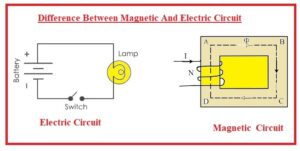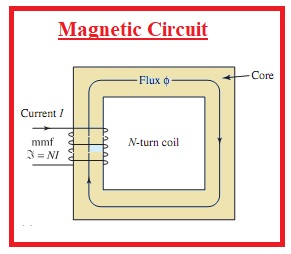Hello, readers welcome to new post. Here we will discuss Difference Between Magnetic And Electric circuits. There are different paramerts related to these two terms and are covered for finding the differences between these two terms such as electromotive force, MMF, current flux etc.

Here we will cover all details about these two terms and their different related factors. So let get started.

# Difference Between Magnetic And Electric Circuit

### What is Electric CIrcuit

• An electric circuit is a path used for the movement of current. This circuit path consists of a device for energy production like battery generators.
• With power devices also consist of different types of loads like a lamp, electric motor, etc., and wires for current flow.• The working of electric circuits depends on the two basic principles of ohms and Kirchhoff laws.
• There are different ways used for describing of electric circuits. Dc current flow in a single direction and AC flow in back and forth movements different appliances used in our houses work on AC.
• In this circuit equation for current and resistance is Current =volts/resistance
• The current measuring unit for this circuit is ampers
• Current flows through electrons
• The series circuit has a path through which current flows in each component connected.
• In a parallel circuit different branches exit and current dividers for each path
• In parallel combination volts about each component is similar and series path current is same

### What is Magnetic CIrcuit

• The closed path that has a magnetic field denoted as magnetic flux is called a magnetic circuit.
• As current flows in the electric circuit, there is no moveable things in the magnetic circuit
• In the case of ring configured electromagnet having small air gap flux retained in the  core and air gap makes the magnetic circuit
• In the motor magnetic field is enclosed in the poles rote and air gaps are among the rotor and poles.
• Its equation is Flux = mmf/reluctance
• Uit used for flux measuring is weber
• Magnetic flux is like the electric current. The magnetomotive force is analogous to emf.• In the case of magnetic circuits, molecular pols have aligned arrangements flow not flow but created in the circuit.

That is all about the Difference Between Magnetic And Electric Circuit all details has been explained. If you have any further queries ask in comments. Thanks for reading have a good day see you in next post.